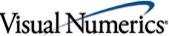# JMSL Numerical Library## Documentation

### Important Notes

Use of JMSL requires a Java development environment, such as Sun's JDK 1.4. Applets built using JMSL are best run using the Java Plug-In, version 1.4.2 or later.

For Windows-based systems, the user may experience different results between OpenGL and Direct 3D (D3D) print output for JMSL 3D graphs. These differences may be related to differences in video hardware and drivers, as wells as versioning issues of OpenGL and D3D. The user should experiment with each method to determine the optimal method for printing 3D graphs. The default is to use OpenGL. To use D3D set the Java system property j3d.rend to d3d. This can be done on the command line using the option -Dj3d.rend=d3d.

Serialization of JMSL classes will not be compatible with future JMSL releases. The current serialization support is appropriate for short term storage or RMI between applications running the same version of JMSL.

This product includes software developed by the Apache Software Foundation (http://www.apache.org). This applies only to the Crimson XML Parser.

## Classes and Methods New to the JMSL Numerical Library Version 4.0

 `com.imsl.math` Optimization `DenseLP` Solves a linear programming problem using an active set strategy. `com.imsl.stat` Basic Statistics `EmpiricalQuantiles` Computes empirical quantiles. Regression `LinearRegression.CaseStatistics` Allows for the computation of predicted values, confidence intervals, and diagnostics for detecting outliers and cases that greatly influence the fitted regression. Probability Distribution Functions and Inverses `Cdf.logNormal` Evaluates the standard lognormal cumulative probability distribution function. `Cdf.inverseLogNormal` Returns the inverse of the standard lognormal cumulative probability distribution function. `Cdf.logNormalProb` Evaluates the standard lognormal probability density function. `Cdf.FProb` Evaluates the F probability density function. `cdf.Weibull` Evaluates the Weibull cumulative probability distribution function. `Cdf.inverseWeibull` Returns the inverse of the Weibull cumulative probability distribution function. `Cdf.WeibullProb` Evaluates the Weibull probablity density function. `Cdf.Rayleigh` Evaluates the Rayleigh cumulative probability distribution function. `Cdf.inverseRayleigh` Returns the inverse of the Rayleigh cumulative probability distribution function. `Cdf.RayleighProb` Evaluates the Rayleigh probablity density function. `Cdf.extremeValue` Evaluates the extreme value cumulative probability distribution function. `Cdf.inverseExtremeValue` Returns the inverse of the extreme value cumulative probability distribution function. `Cdf.extremeValueProb` Evaluates the extreme value probability density function. `Cdf.betaProb` Evaluates the beta probablity density function. `Cdf.gammaProb` Evaluates the gamma probability density function. `Cdf.exponential` Evaluates the exponential cumulative probability distribution function. `Cdf.inverseExponential` Evaluates the inverse of the exponential cumulative probability distribution function. `Cdf.exponentialProb` Evaluates the exponential probability density function. `Cdf.geometric` Evaluates the discrete geometric cumulative probability distribution function. `Cdf.geometricProb` Evaluates the discrete geometric probability density function. `Cdf.inverseGeometric` Returns the inverse of the discrete geometric cumulative probability distribution function. `Cdf.chiProb` Evaluates the chi-squared probablity density function. `Cdf.noncentralchi` Evaluates the noncentral chi-squared cumulative probability distribution function. `Cdf.inverseNoncentralchi` Evaluates the inverse of the noncentral chi-squared cumulative probability distribution function. `Cdf.noncentralstudentsT` Evaluates the noncentral Student's t cumulative probability distribution function. `Cdf.inverseNoncentralstudentsT` Evaluates the inverse of the noncentral Student's t cumulative probability distribution function. `Cdf.bivariateNormal` Evaluates the bivariate normal cumulative probability distribution function. `Cdf.discreteUniform` Evaluates the discrete uniform cumulative probability distribution function. `Cdf.inverseDiscreteUniform` Returns the inverse of the discrete uniform cumulative probability distribution function. `Cdf.discreteUniformProb` Evaluates the discrete uniform probability density function. `Cdf.uniform` Evaluates the uniform cumulative probability distribution function. `Cdf.inverseUniform` Returns the inverse of the uniform cumulative probability distribution function. Random Number Generation `Random.nextExtremeValue` Generate a pseudorandom number from an extreme value distribution. `Random.nextF` Generate a pseudorandom number from the F distribution. `Random.nextRayleigh` Generate a pseudorandom number from a Rayleigh distribution. `MersenneTwister` Initializes the 32-bit Mersenne Twister generator. `MersenneTwister64` Initializes the 64-bit Mersenne Twister generator. `com.imsl.io` Input/Output `MPSReader` Reads a linear programming problem from an MPS file. `com.imsl.chart` Chart 2d `Dendrogram` A dendrogram chart for cluster analysis. `com.imsl.chart3d` Chart 3d `AmbientLight` An ambient light. `Axis3D` An x-axis, y-axis or a z-axis. `AxisBox` Box behind the axis. `AxisLabel` The labels on an axis. `AxisLine` The axis line. `AxisTitle` Axis title. `AxisXYZ` The axis for an x-y-z chart. `Background` Background of the chart. `BufferedPaint` A paint object cached into an image image on the canvas. `Canvas3DChart` A canvas for rendering a 3d chart. `Chart3D` Root node of a 3d chart tree. `ChartLights` Default set of lights. `ChartNode3D` The base class of all of the nodes in the 3D chart tree. `ColormapLegend` Adds a legend for a Colormap gradient to the background of the canvas. `Data` Draws a 3D data node. `DirectionalLight` A directional light. `JFrameChart3D` JFrameChart3D is a JFrame that contains a chart. `MajorTick` The major tick marks. `PointLight` A point light source. `Surface` Surface from a function or from a set of scattered data points. `com.imsl.datamining.neural` Neural Nets `BinaryClassification` Classifies patterns into two classes. `MultiClassification` Classifies patterns into three or more classes. `FeedForwardNetwork.setEqualWeights` Initialize network weights using equal weighting. `FeedForwardNetwork.setRandomWeights` Initialize network weights using random weighting.

## Changes in JMSL Numerical Library Version 4.0

 `com.imsl.math` Tranforms `FFT` Corrected the equations in the documentation. Optimization `MinUnconMultiVar` Add code to prevent a divide by zero condition. `com.imsl.stat` Basic Statistics `Anova.getP` Corrected the problem where 0 was being returned for very small values. Time Series and Forecasting `ARMA.setBackwardOrigin` Error checking was added to avoid an out of bounds exception. `Anova.getDunnSidak` Clarify documentation on the description of the return value. Regression `SelectionRegression` Return an error when the mean squared error for the full model is near zero. This avoids bad Cp values being returned. ` ` A check was added to prevent an out of bounds exception. Random Number Generation `Random.nextGeometric` Added an error message to signal when the input arguments are out of range. `Random.nextLogarithmic` Added an error message to signal when the input arguments are out of range. Categorical and Discrete Data Analysis `CategoricalGenLinModel` Increase internal workspace arrays to avoid an out of memory error. `com.imsl.finance` Finance `Bond.duration` Corrected the initialization of a local variable in the duration calculation. `npv` An IllegalArgumentException has been added when rate == -1. `irr` Implemented scaling of large input arguments to avoid incorrect results. `com.imsl.chart` Chart 2d `JFrameChart` Corrected the class description in the documentation.